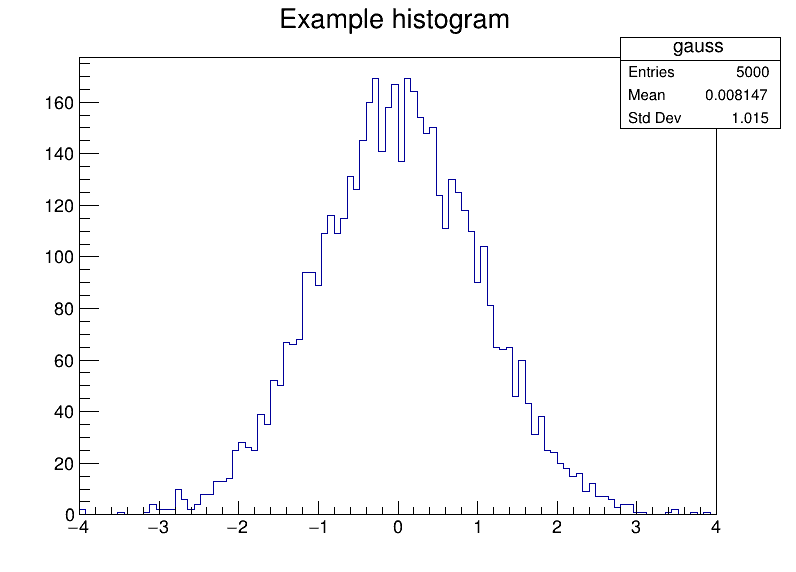# How to use ROOT in a Jupyter notebook¶

ROOT is integrated with the Jupyter notebook technology. There are two alternatives for using ROOT in a notebook:

1. Python flavour: the default language of the notebook is Python and ROOT is accessed via the PyROOT interface. The user can mark cells to be C++ with the %%cpp magic.
2. C++ flavour: the notebook is entirely written in C++, thus emulating the ROOT C++ prompt.

This Python notebook will show how to use the Python flavour.

## Python flavour¶

In order to use ROOT in a Python notebook, we first need to import the ROOT module. During the import, all notebook related functionalities are activated.

In :
import ROOT

Welcome to JupyROOT 6.18/00


Now we are ready to use PyROOT. For example, we create a histogram.

In :
h = ROOT.TH1F("gauss","Example histogram",100,-4,4)
h.FillRandom("gaus")


Next we create a canvas, the entity which holds graphics primitives in ROOT.

In :
c = ROOT.TCanvas("myCanvasName","The Canvas Title",800,600)
h.Draw()


For the histogram to be displayed in the notebook, we need to draw the canvas.

In :
c.Draw()It is not active by default yet, but Javascript visualisation can be activated for testing purposes. The plot below will be interactive: click on it and discover the JSROOT capabilities!

In :
%jsroot on
c.Draw()


### Interleave Python with C++: the %%cpp magic¶

Thanks to ROOT, it is possibile to write cells in C++ within a Python notebook. This can be done using the %%cpp magic. Magics are a feature of Jupyter notebooks and when importing the ROOT module, the %%cpp magic was registered.

In :
%%cpp
cout << "This is a C++ cell" << endl;

This is a C++ cell


Not bad. On the other hand, ROOT offers much more than this. Thanks to its type system, entities such as functions, classes and variables, created in a C++ cell, can be accessed from within Python.

In :
%%cpp
class A{
public:
A(){cout << "Constructor of A!" << endl;}
};

In :
a = ROOT.A()

Constructor of A!


The Python and C++ worlds are so entangled that we can find back in C++ the entities created in Python. To illustrate this, from within a C++ cell, we are going to fit a function in the gauss histogram displayed above and then re-draw the canvas.

In :
%%cpp
gauss->Fit("gaus", "S");
myCanvasName->Draw();

 FCN=65.0502 FROM MIGRAD    STATUS=CONVERGED      54 CALLS          55 TOTAL
EDM=9.21369e-07    STRATEGY= 1      ERROR MATRIX ACCURATE
EXT PARAMETER                                   STEP         FIRST
NO.   NAME      VALUE            ERROR          SIZE      DERIVATIVE
1  Constant     1.57425e+02   2.76819e+00   8.89596e-03  -5.39870e-04
2  Mean         1.38321e-02   1.43799e-02   5.69927e-05   8.22299e-04
3  Sigma        1.00178e+00   1.04857e-02   1.11224e-05  -4.31183e-01


Complete interoperability is possible. Let's move now to the options offered by the %%cpp magic.

### The options of the %%cpp magic¶

The %%cpp magic accepts two options: -d and -a. Their documentation can be seen by typing:

In :
%%cpp?


A window will appear at the bottom of the page, showing the documentation.

#### ACliC¶

The first option (-a) allows to compile the cell code with ACLiC. This is not so relevant for performance since the ROOT interpreter just in time compiles the C++ code. Nevertheless, ACLiC is most useful when the automatic creation of dictionaries is required, for example in presence of I/O operations.

In :
%%cpp -a
class CompileMe {
public:
CompileMe() {}
void run() {}
};

Info in <TUnixSystem::ACLiC>: creating shared library /home/myuser/64a4a5eb_C.so


Let's verify that the dictionary is there:

In :
ROOT.TClass.GetClass("CompileMe").HasDictionary()

Out:
True

Note that the previously created class A has no dictionary.

In :
ROOT.TClass.GetClass("A").HasDictionary()

Out:
False

#### Declaration of functions¶

The second option (-d) needs to be used when declaring functions. The interpreter cannot yet detect function declarations by itself: we need to be explicit. This is a limitation which will be lifted in the near future.

In :
%%cpp -d
void f() {
cout << "This is function f" << endl;
}


As usual, function f can also be accessed from Python.

In :
print "This is again Python"
ROOT.f()

This is again Python
This is function f